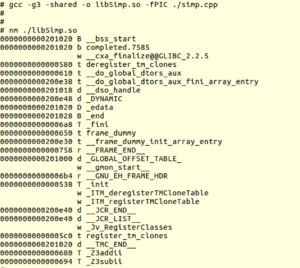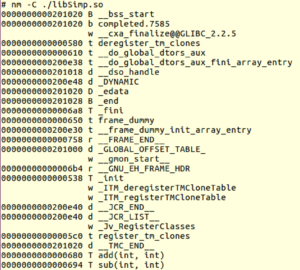While writing applications in linux , the developer can use tons of libraries. Each library can packs functions, classes and variables and it is very  important to understand how to create and work with it.

```int add(int a,int b)
{
return a+b;
}

int sub(int a,int b)
{
return a-b;
}
```

To compile the library we use:

`# gcc -g3 -shared -o libSimp.so -fPIC ./simp.c`

Here we use -g3 to add debugging information , -shared to specify that we build a shared object and -fPIC to specify that this build a position independent code (we don’t know where in the memory the shared library will be loaded)

Using the library:

```#include<stdio.h>

#include "simp.h"

void main()
{
int a,b;
b=sub(80,20);
printf("a=%d b=%d\n",a,b);
}```

To build the application we need to use -l with the library name and -L to specify the library location. If we put the library in one of the lib directories (/lib , /usr/lib) we don’t need to specify the location using -L flag but usually we want to put our code in a separate place

`# gcc -g3 -o simp -L . usesimp.c -lSimp`

Here we use -L with the current directory. note that don’t write the lib prefix and the extension so any library should named libXXX.so and to link with it we specify -lXXX

To run the app we need to put the library in one of the path locations or specify the library path use LD_LIBRARY_PATH environment variable:

```# export LD_LIBRARY_PATH=.
# ./simp
a=30 b=60
```

What if we don’t know on build time the name of the loaded library ? For example we want to build an app that support plugins – for each plugin the user should implement 2 functions that we specify the prototype for. In this case we can use dlopen(3)

We build the library the same way , the different is the application using it:

```#include<stdio.h>
#include<stdlib.h>
#include<dlfcn.h>

int main()
{
int (*fptr)(int,int);
void *libh=dlopen("./libSimp.so",RTLD_LAZY);
if(libh == NULL)
{
exit(0);
}
if(fptr == NULL)
{
puts("Error finding function");
exit(0);
}
printf("sum = %d\n",fptr(20,10));
dlclose(libh);
return 0;
}
```

To compile it use:

`gcc -o usedlopen ./usedlopen.c -ldl`

We need to add -ldl to use dlopen.

Note that we are using the symbol name. In C the function name is also the symbol name but if we compile it to c++ as a result of function overloading the symbol name is different. To see the symbols , use the nm utility:As you can see in the last 2 lines the symbol names are different because the compiler decode also the function prototype so if we want to use the above example with this library we need to use the correct symbol name:

`fptr=dlsym(libh,"_Z3addii");`

Another option is to add extern “C” on the library functions prototype to make the compiler use the name as a symbol name.

If we can’t find the function name we can ask nm to display the functions prototype using -C :Now we can see the functions, using their address we can find the symbol name

#### Global Variables

One basic concept of any general purpose operating system is the private process address space – means each process has its on private memory space and the system maps pages from the physical address space to virtual for every process. Shared libraries are code sharing mechanism and not data means that every process has its own copy for example if we add a global variable to our library:

```int add(int a,int b)
{
return a+b;
}

int global=100;

int sub(int a,int b)
{
return a-b;
}
```

and we use it by 2 separate processes :

```/* simp.h */
#ifndef SIMPLIB
#define SIMPLIB

int sub(int a,int b);
extern int global;

#endif

/*****************************/
/* simp.c */
#include<stdio.h>
#include "simp.h"

void main()
{
int a,b;
b=sub(80,20);
printf("a=%d b=%d global=%d\n",a,b,global);
global=200;
sleep(100);
}

/*****************************/
/* simp2.c */
#include<stdio.h>

#include "simp.h"

void main()
{
printf("global=%d\n",global);
sleep(100);
}```

If you run simp.c and wait until it gets to sleep so global=200 and then run simp2.c you will get global = 100

To create a shared data we need to use shared memory (shm_open)

First we define a structure to hold our data – Do Not Use Pointers !!! :

```/* simp.h */
#ifndef SIMPLIB
#define SIMPLIB

int sub(int a,int b);

struct shared_data{
int data1;
char buf;
int num;
};

extern struct shared_data *global;

#endif```

Then we create a shared memory on library load (note the constructor attribute to declare a function as initializer)

```#include <sys/mman.h>
#include <sys/stat.h>
#include <fcntl.h>
#include <unistd.h>

#include "simp.h"

static void __attribute__ ((constructor)) init_lib(void);

struct shared_data *global;

void init_lib(void)
{
int fd=shm_open("/libmem", O_RDWR | O_CREAT , 0660);
ftruncate(fd,sizeof(struct shared_data));
}

{
return a+b;
}

int sub(int a,int b)
{
return a-b;
}
```

Now if we create 2 or more processes accessing global , the data will be shared

This example is for learning purpose just to show a simple way to share data, If you do that for real application it will be better to hide the structure, define a set of getters/setters and check on init if this is the first process loading to library so we can initialize the data.

To initialize the shared memory on the first instance creating it use O_EXCL flag in shm_open

```#include <sys/mman.h>
#include <sys/stat.h>
#include <fcntl.h>
#include <unistd.h>

#include "simp.h"

static void __attribute__ ((constructor)) init_lib(void);

struct shared_data *global;

void init_lib(void)
{
int fd=shm_open("/libmem", O_RDWR | O_CREAT | O_EXCL, 0660);
if(fd == -1)
{
sleep(1); // wait just in case the other instance didnt finish the initialization
fd=shm_open("/libmem", O_RDWR, 0);
}
else
{
ftruncate(fd,sizeof(struct shared_data)); // set the size
global->data1 = 100; // some init examples
global->num= 50;
}

}

{
return a+b;
}

int sub(int a,int b)
{
return a-b;
}
```

If we want to delete the shared memory while no process is mapped we can also implement destructor add add a reference count + mutex in the shared  memory

Tagged ,

## 1 thought on “Linux Shared Libraries”

1. […] can build the fault handler into a shared library and use the constructor to set it. Then you can inject the library to any process using […]